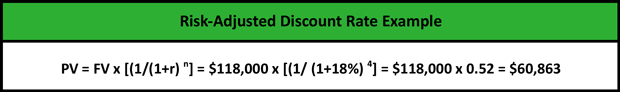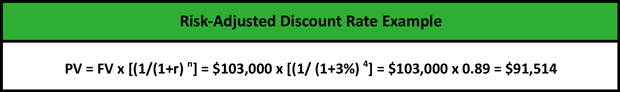# What is a Risk-Adjusted Discount Rate?

Definition: Risk-adjusted discount rate is the rate used in the calculation of the present value of a risky investment, such as the real estate or a firm. In fact, the risk-adjusted discount rate represents the required return on investment.

## What Does Risk Adjusted Discount Rate Mean?

What is the definition of risk adjusted discount rate? The risk-adjusted discount rate is the total of the risk-free rate, i.e. the required return on risk-free investments, and the market premium, i.e. the required return of the market. Financial analysts use the risk-adjusted discount rate to discount a firm’s cash flows to their present value and determine the risk that investor should accept for a particular investment.

The difference between the market premium, which is often used as a discount rate in valuation analysis is that the risk-adjusted discount rate takes into consideration the future market conditions, the level of inflation and the value of money at the end of the investment horizon.

Let’s look at an example.

## Example

A leading technology company is considering undertaking a 4-year project that requires an invested capital of \$100,000 and is expected to return 18% or \$118,000 at maturity.

The present value of the project is:Since the present value of the expected cash flows is lower than the invested capital, the manager wants to calculate the present value of the expected cash flows using the risk-adjusted discount rate 3% that will generate \$103,000 and reflects all the risks involved.

The present value of the project using the risk-adjusted discount rate is:By using the risk-adjusted discount rate, the present value of the expected cash flows is almost equal to the invested capital of \$100,000. However, with the adjustment of the discount rate to reflect all the risks of the project, the present value of cash flows is still lower than the invested capital. Therefore, the project should not be undertaken.

## Summary Definition

Define Risk-Adjusted Discount Rate: RADR is a financial measurement used to calculate the present value of an investment with a high degree of risk.